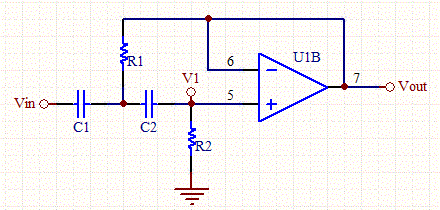# Sallen-Key High Pass Butterworth Filter CalculatorThis calculator calculates the capacitor values for a unity gain Sallen-Key high pass Butterworth filter. The Sallen-Key filter is a simple active filter based on op-amps stages, which is ideal for filtering audio frequencies.

For a derivation of the design equations see this link:

To use this calculator, select a resistance, the number of poles, and the cut-off frequency.

 3dB Frequency (Hz) Capacitor (C1,C2=C) (uF) Poles: 2 4 6 8 10

R1= A/(4*C*PI*f)

R2= 1/(A*C*PI*f)

### Derivation:

If normalized then Wo=1 and Q= 1/A. (See the design equation page for R1 and R2)